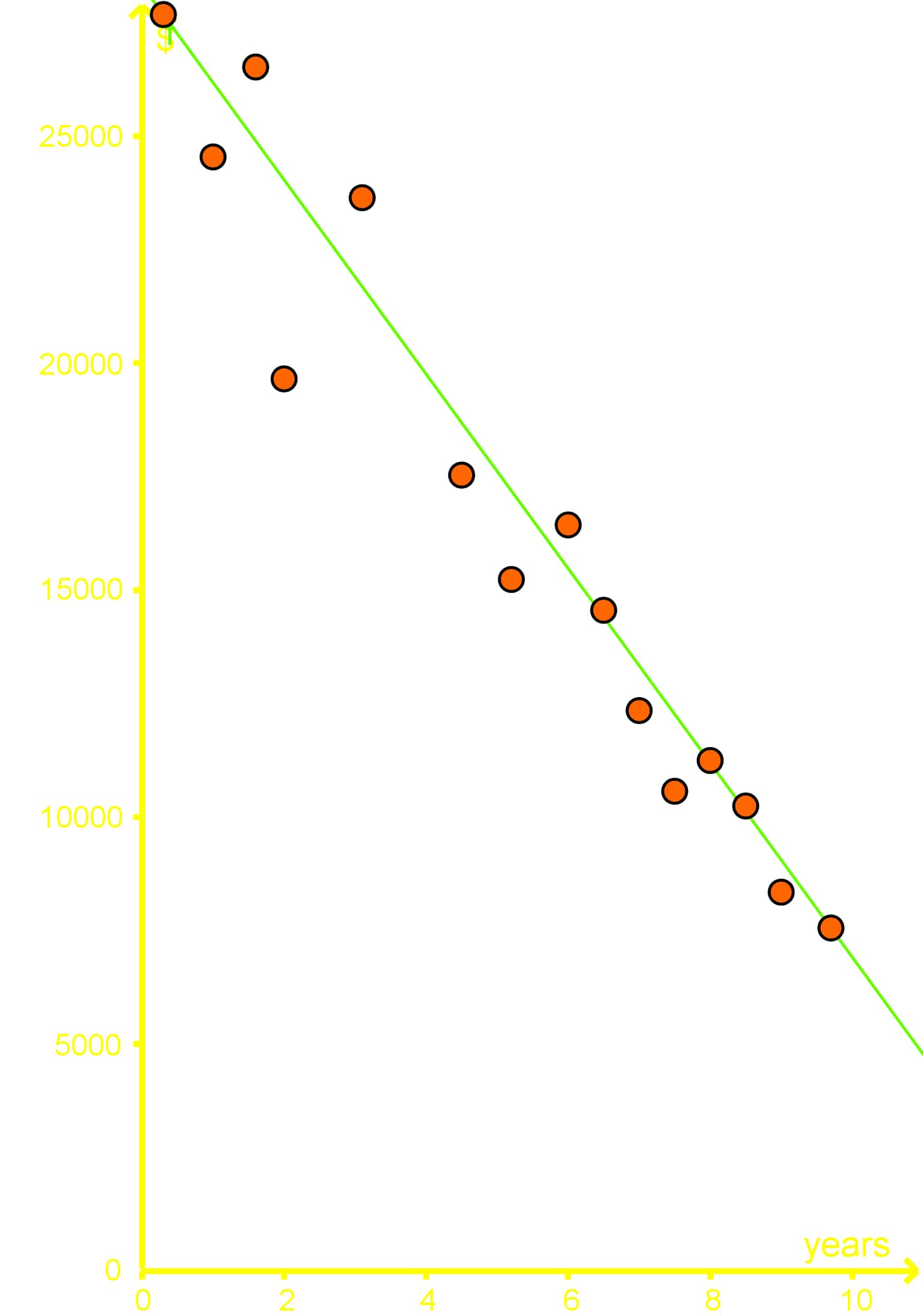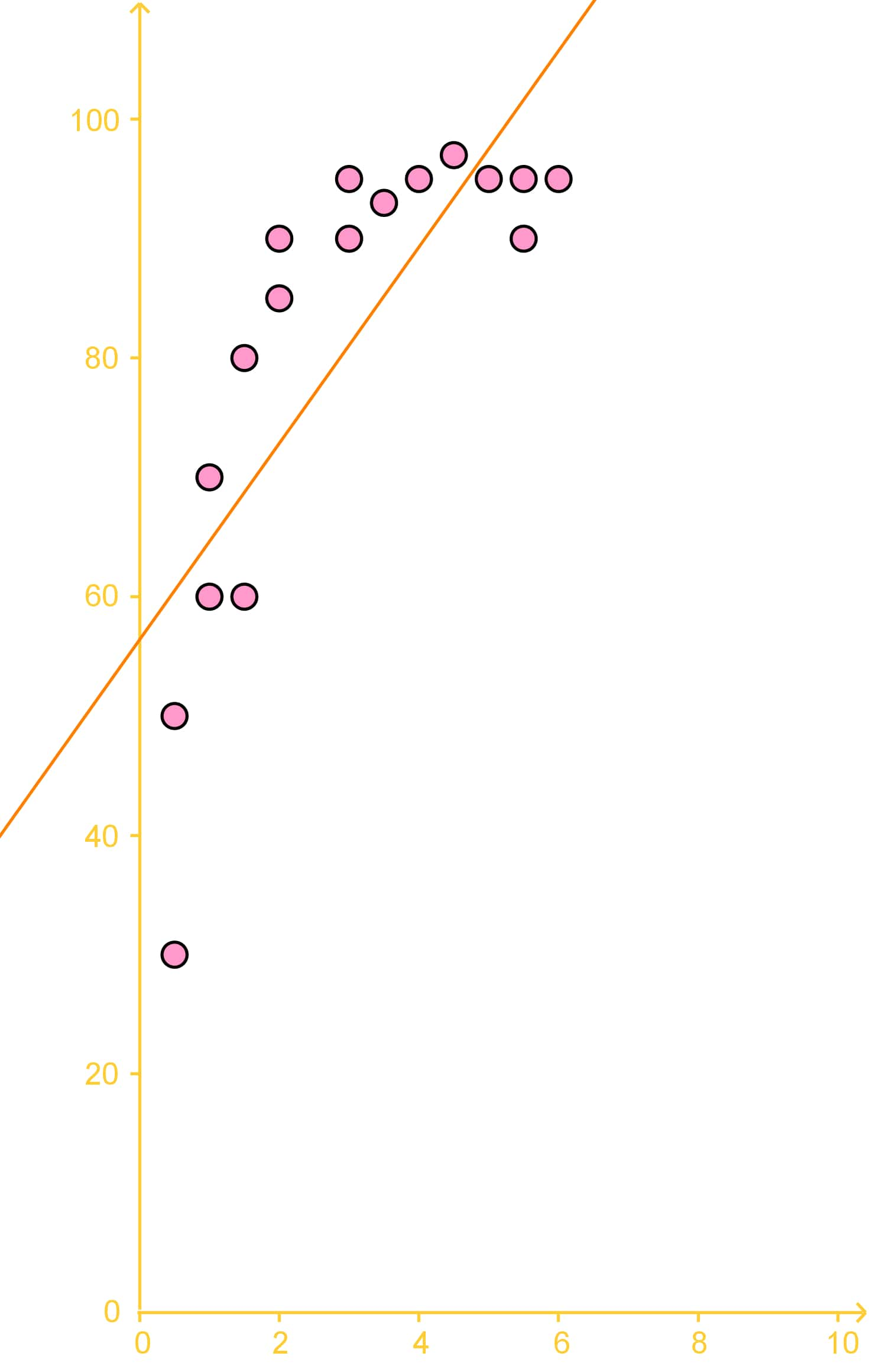# Regression analysis

### Regression analysis

#### Lessons

Regression analysis is what we call our estimation of our bivariate data
• The simplest model for regression analysis is a "line of best fit" or a "trend line"
Interpolation is our estimation of finding a new data point that lies within our known set of data points.
Extrapolation is our estimation of a new data point that lies outside our known set of data points.
• Introduction
What is Regression Analysis?

• 1.
Interpolation and Extrapolation
A study took a sample of 14 cars and found the age of each car and the amount of money that each car is worth. A best fit line is given by the equation $y=-2100x+27500$, where y is the worth of the car in dollars and $x$ is the age of the car in years.a)
Using the best fit line interpolate what a car might be worth if it was 5 years old?

b)
Using extrapolation what might a car be worth if it was 11 years old?

c)
Using extrapolation what might a car be worth if it was 15 years old? What's wrong with this answer?

• 2.
A study was done on 17 factory workers on their coffee consumption and how many boxes they were able to move per hour. The results of this study are given in the graph below:The trend line is given as: $y=8x+56$, with $y$ being the number of boxes moved per hour and $x$ being the number of coffees drunk.
a)
Using the trend line extrapolate how many boxes would be moved if a worker were to drink 15 coffees?

b)
Is the extrapolation done in the previous part a good estimate? Why or why not?

• 3.
Finding the Best Fitting Curve
a)
Plot the following bivariate data as a scatter plot, with the time spent on homework on the x-axis.
 Time Spent on Homework Grade in Class 2 hours 65% 8 hours 80% 13 hours 83% 15 hours 87% 19 hours 91% 24 hours 93%

b)
Make a line of best fit using the student who spent 8 hours on homework and the student who spent 15 hours studying

c)
Using the best fit line found in the previous part, estimate what mark a student would achieve in this class if they spent 14 hours on homework